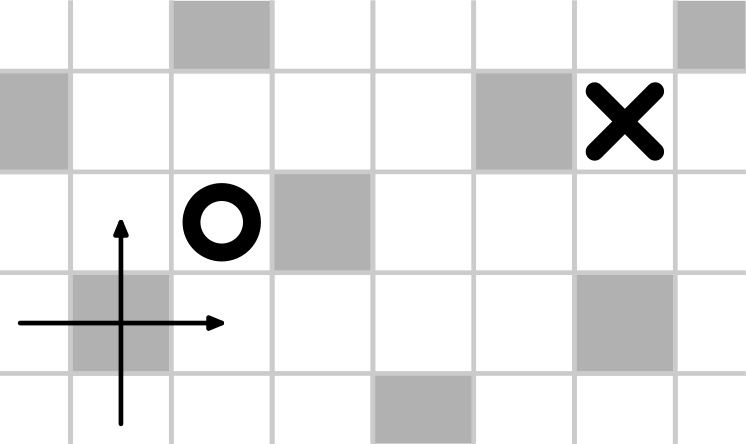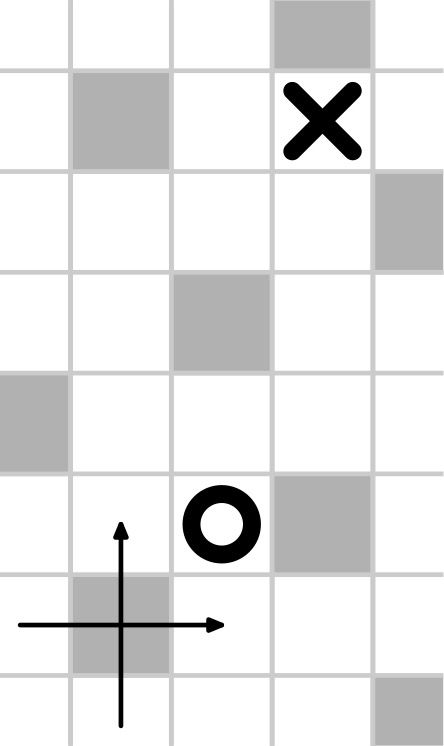시간 제한메모리 제한제출정답맞힌 사람정답 비율
2 초 (추가 시간 없음) 256 MB0000.000%

## 문제

Consider a plane partitioned into squares with side $1$. Let us choose a square and draw coordinate axes from its center parallel to its sides.

Each fifth square contains an obstacle: more precisely, the obstacles are placed in all squares with centers at points $(2 i + j, i - 2 j)$ for all possible integer $i$ and $j$. The exact placement of obstacles is visualized in the example notes below. All other squares are free.

Amelia stands in some free square $A$ and wants to move to some free square $B$. In one step, she can move from a square to one of its neighbors: the squares sharing a side with it, but only if the corresponding neighboring square is free. What is the minimum possible number of steps Amelia must make to arrive to square $B$?

## 입력

The first line contains two integers $x_1$ and $y_1$, the coordinates of the initial square $A$. The second line contains two integers $x_2$ and $y_2$, the coordinates of the destination square $B$. All given coordinates are between $1$ and $10^{9}$. It is guaranteed that both given squares are free.

## 출력

Print one integer: the minimum possible number of steps from the initial square to the destination square.

## 예제 입력 1

1 1
5 2


## 예제 출력 1

7## 예제 입력 2

1 1
2 5


## 예제 출력 2

5